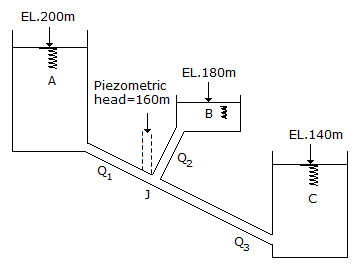# Civil Engineering - GATE Exam Questions - Discussion

### Discussion :: GATE Exam Questions - Section 2 (Q.No.38)

38.

Three reservoirs A, B and C are interconnected by pipes as shown in the below figure Water surface elevations in the reservoirs and the Piezometric Head at the junction J are indicated in the below figure.Discharges Q1, Q2 and Q3 are related as

 [A]. Q1 + Q2 = Q3 [B]. Q1 = Q2 + Q3 [C]. Q2 = Q1 + Q3 [D]. Q1 + Q2 + Q3 = 0

Explanation:

No answer description available for this question.

 Venkatesh said: (Jan 4, 2014) If we take datum at bottom of tank C we conclude that tanks A, B, and C have heads 200m, 180m, 140m respectively but at junction we have some elevation head w.r.t datum and in addition piezometric head of 160m given which may be more than total heads of B, and C so water flows from A to B and C.

 Khatana said: (Dec 13, 2016) No, when head at junction-less than B then how it will fill B option A?

 Radhika said: (Nov 22, 2017) Flow always takes place from higher energy level to the lower level. Hence [B] is correct.

 Yogesh said: (Jun 10, 2018) Option A is correct. Q1 takes place from 200 to J (160), Q2 takes place from 180 to J (160), Q3 takes place from J (160) to 140. So Q3 = Q1 + Q2.

 Saini said: (Jul 29, 2019) Option A is correct. I also agree.

 Dipesh said: (Aug 19, 2019) But piezometer level only shows p/(density)g + z. It doesn't show v^2/2g. So it's not total head then how can we make judgement without velocity head?

 Abhishek Raaz said: (Jan 22, 2020) At junction point J velocity head will be zero so total head at J will be 160 m. The total head at A=200m and total head at B=180m and total head at C=140 m. Now you can judge flow condition from higher energy levels to lower levels.

 Niladri Roy said: (Mar 1, 2020) Q1+Q2=Q3.

 Sneha Mathai said: (Dec 10, 2020) Ans is option A. Flow takes place from higher energy level to lower energy level. Head for A, 200m > head at jn, 160m So flow from A to jn. Head for B, 180m > head at jn, 160m So flow from B to jn. Head for C, 140m < head at jn, 160m So flow from jn to C. Hence, Q1 + Q2 = Q3.